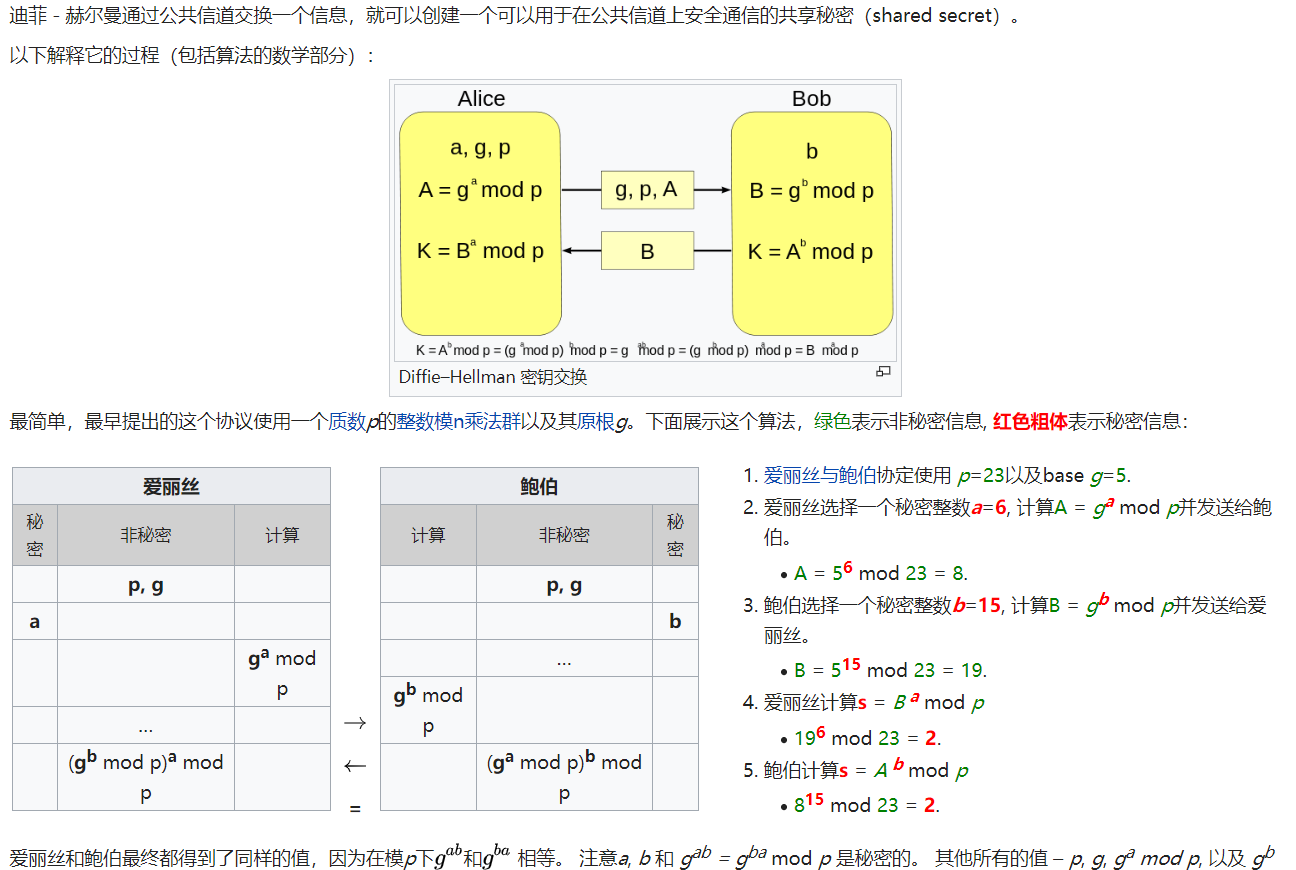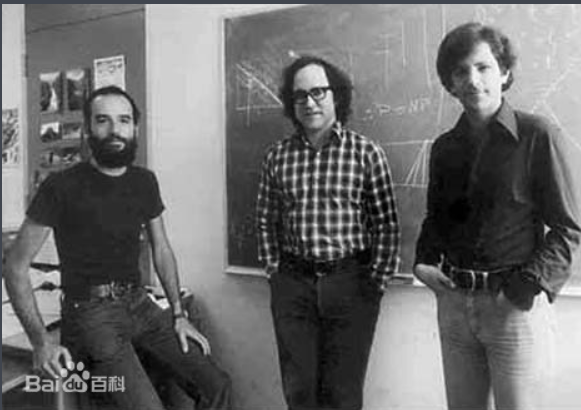# The idea and origin of public key encryption

## 对称加密

1976年,在一篇名叫密码学的新方向的论文中,Whitefield与Martin Hellman首次提到了一种解决方法.该方法被称为Diffie-Hellman密钥协商算法($Diffie-Hellman Key Exchange/Agreement Algorithm$).DH密钥协商主要解决秘钥配送问题，本身并非用来加密用的；该算法其背后有对应数学理论做支撑，简单来讲就是构造一个复杂的计算难题，使得对该问题的求解在现实的时间内无法快速有效的求解（computationally infeasible)

## 灵光一闪

1970年，一位名叫James Ellis的密码学家为英国政府通信总部工作,在wiki百科中有一段话写道:

Ellis said that the idea first occurred to him after reading a paper from World War II by someone at Bell Labsdescribing the scheme named Project C43, a way to protect voice communications by the receiver adding (and then later subtracting) random noise

## 简单原理

1973年,在英国政府通讯总部工作的数学家克利福德·柯克斯(Clifford Cocks)提出了这样算法

$m^{e}\bmod N = c \\ c^{d}\bmod N= m$

$(m^{e})^{d}\bmod N= m$

$m^{\varphi(n)}\equiv1\bmod N$

$m^{k*{\varphi(n)}}\equiv1\bmod N$

$m^{k*{\varphi(n)+1}}\equiv m\bmod N$

$ed= k*\varphi(n)+1 \Rightarrow ed\equiv 1 \bmod \varphi(n)$

$d= \frac {k*\varphi(n)+1}{e}$